Courses

# Introduction To Signals

## 10 Questions MCQ Test Mock Test Series for Electrical Engineering (EE) GATE 2020 | Introduction To Signals

Description
This mock test of Introduction To Signals for GATE helps you for every GATE entrance exam. This contains 10 Multiple Choice Questions for GATE Introduction To Signals (mcq) to study with solutions a complete question bank. The solved questions answers in this Introduction To Signals quiz give you a good mix of easy questions and tough questions. GATE students definitely take this Introduction To Signals exercise for a better result in the exam. You can find other Introduction To Signals extra questions, long questions & short questions for GATE on EduRev as well by searching above.
QUESTION: 1

Solution:
QUESTION: 2

Solution:
QUESTION: 3

### The auto-correlation function Rx(τ) satisfies which one of the following properties?

Solution:
QUESTION: 4

The auto-correlation function Rx(τ) of the signal x(t) = V sinωt is given by

Solution:
QUESTION: 5

The sampling of a function f(t) = sin(2πf0t) starts from zero-crossing. The signal can be detected, if sampling time T is

Solution:
QUESTION: 6

What is the power and energy of the unit step sequence u(n) respectively?

Solution:
QUESTION: 7

Let δ(f) is the delta function the value of integral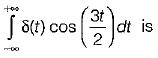Solution:
QUESTION: 8

If a signal f(t) has energy ‘E’ the energy of the signal f(2t) is equal to

Solution:and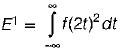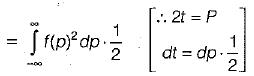⇒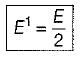QUESTION: 9

Consider the sequence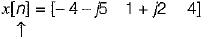the conjugate anti-symmetric part of the sequence is

Solution: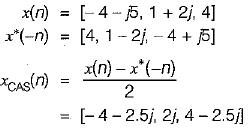QUESTION: 10

The function x(t) is shown in the figure. Even and odd parts of a unit step function u(t) are respectively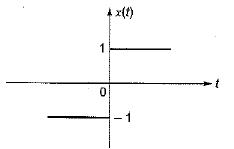Solution: